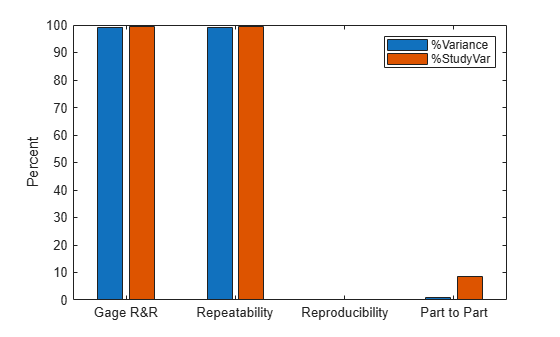gagerr

Gage repeatability and reproducibility study

Syntax

gagerr(y,{part,operator})
gagerr(y,GROUP)
gagerr(y,part)
gagerr(...,param1,val1,param2,val2,...)
[TABLE, stats] = gagerr(...)

Description

gagerr(y,{part,operator}) performs a gage repeatability and reproducibility study on measurements in y collected by operator on part. y is a column vector containing the measurements on different parts. part and operator are categorical variables, numeric vectors, character matrices, string arrays, or cell arrays of character vectors. The number of elements in part and operator should be the same as in y.

gagerr prints a table in the command window in which the decomposition of variance, standard deviation, study var (5.15 x standard deviation) are listed with respective percentages for different sources. Summary statistics are printed below the table giving the number of distinct categories (NDC) and the percentage of Gage R&R of total variations (PRR).

gagerr also plots a bar graph showing the percentage of different components of variations. Gage R&R, repeatability, reproducibility, and part-to-part variations are plotted as four vertical bars. Variance and study var are plotted as two groups.

To determine the capability of a measurement system using NDC, use the following guidelines:

• If NDC > 5, the measurement system is capable.

• If NDC < 2, the measurement system is not capable.

• Otherwise, the measurement system may be acceptable.

To determine the capability of a measurement system using PRR, use the following guidelines:

• If PRR < 10%, the measurement system is capable.

• If PRR > 30%, the measurement system is not capable.

• Otherwise, the measurement system may be acceptable.

gagerr(y,GROUP) performs a gage R&R study on measurements in y with part and operator represented in GROUP. GROUP is a numeric matrix whose first and second columns specify different parts and operators, respectively. The number of rows in GROUP should be the same as the number of elements in y.

gagerr(y,part) performs a gage R&R study on measurements in y without operator information. The assumption is that all variability is contributed by part.

gagerr(...,param1,val1,param2,val2,...) performs a gage R&R study using one or more of the following parameter name/value pairs:

• 'spec' — A two-element vector that defines the lower and upper limit of the process, respectively. In this case, summary statistics printed in the command window include Precision-to-Tolerance Ratio (PTR). Also, the bar graph includes an additional group, the percentage of tolerance.

To determine the capability of a measurement system using PTR, use the following guidelines:

• If PTR < 0.1, the measurement system is capable.

• If PTR > 0.3, the measurement system is not capable.

• Otherwise, the measurement system may be acceptable.

• 'printtable' — A value 'on' or 'off' that indicates whether the tabular output should be printed in the command window or not. The default value is 'on'.

• 'printgraph' — A value 'on' or 'off' that indicates whether the bar graph should be plotted or not. The default value is 'on'.

• 'randomoperator' — A logical value, true or false, that indicates whether the effect of operator is random or not. The default value is true.

• 'model' — The model to use, specified by one of:

• 'linear' — Main effects only (default)

• 'interaction' — Main effects plus two-factor interactions

• 'nested' — Nest operator in part

The default value is 'linear'.

[TABLE, stats] = gagerr(...) returns a 6-by-5 matrix TABLE and a structure stats. The columns of TABLE, from left to right, represent variance, percentage of variance, standard deviations, study var, and percentage of study var. The rows of TABLE, from top to bottom, represent different sources of variations: gage R&R, repeatability, reproducibility, operator, operator and part interactions, and part. stats is a structure containing summary statistics for the performance of the measurement system. The fields of stats are:

• ndc — Number of distinct categories

• prr — Percentage of gage R&R of total variations

• ptr — Precision-to-tolerance ratio. The value is NaN if the parameter 'spec' is not given.

Examples

collapse all

Simulate a measurement system by randomly generating the operators, parts, and the measurements, y , operators do on the parts.

rng(1234,'twister')               % for reproducibility
y = randn(100,1);                 % measurements
part = ceil(3*rand(100,1));       % parts
operator = ceil(4*rand(100,1));   % operators

Conduct a gage R&R study for this system using a mixed ANOVA model without interactions.

gagerr(y,{part, operator},'randomoperator',true)
Columns 1 through 4

{'Source'           }    {'Variance'}    {'% Variance'}    {'sigma' }
{'Gage R&R'         }    {[  0.9715]}    {[   99.2653]}    {[0.9857]}
{'  Repeatability'  }    {[  0.9535]}    {[   97.4201]}    {[0.9765]}
{'  Reproducibility'}    {[  0.0181]}    {[    1.8452]}    {[0.1344]}
{'   Operator'      }    {[  0.0181]}    {[    1.8452]}    {[0.1344]}
{'Part'             }    {[  0.0072]}    {[    0.7347]}    {[0.0848]}
{'Total'            }    {[  0.9787]}    {[       100]}    {[0.9893]}

Columns 5 through 6

{'5.15*sigma'}    {'% 5.15*sigma'}
{[    5.0762]}    {[     99.6320]}
{[    5.0288]}    {[     98.7016]}
{[    0.6921]}    {[     13.5838]}
{[    0.6921]}    {[     13.5838]}
{[    0.4367]}    {[      8.5716]}
{[    5.0949]}    {0x0 char      }

Number of distinct categories (NDC):0
% of Gage R&R of total variations (PRR): 99.63
Note: The last column of the above table does not have to sum to 100%Burdick, Richard K., Connie M. Borror, and Douglas C. Montgomery. Design and Analysis of Gauge R&R Studies: Making Decisions with Confidence Intervals in Random and Mixed ANOVA Models. Society for Industrial Applied Mathematics: American Statistical Association, 2005.